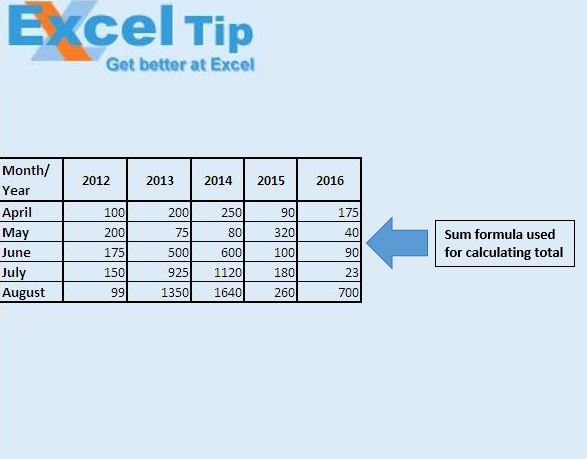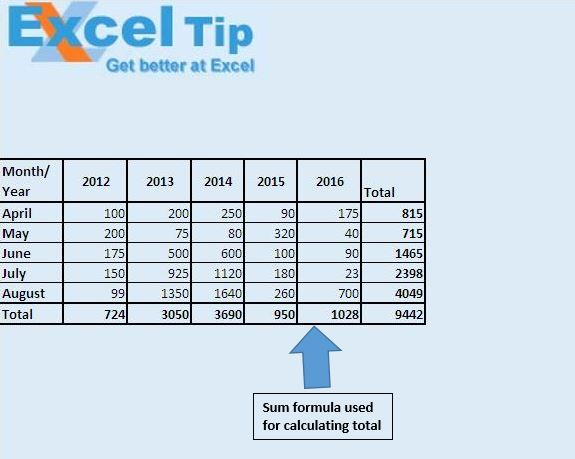# Cell protection only for formulas using VBA in Microsoft Excel

In this article, we will use change event of worksheet to protect cells which contain formulas.

Raw data consists of employee sales data, which includes bifurication by month and year.We want to find out total sales by month, as well as by year.We have used sum function to calculate total sales on monthly and yearly basis. Change event of worksheet is used to protect cells which contain formulas. When we try to edit a cell containing a formula, we will get a pop-up message, saying that the cell value can't be changed.

Code explanation

rng.HasFormula

HasFormula method will return True if a cell contains a formula. Otherwise it will return False.

ActiveSheet.Protect

The above code is used to protect a cell.

Note: A cell will be protected only if it is locked. So before protecting a cell, it must be locked.

ActiveSheet.Unprotect

The above code is used to unprotect a cell.

```'Add below code in worksheet module
Option Explicit

Private Sub Worksheet_SelectionChange(ByVal Target As Range)

Dim rng As Range

For Each rng In Target.Cells
If rng.HasFormula Then
ActiveSheet.Protect
Else
ActiveSheet.Unprotect
End If
Next rng

End Sub

```

We would love to hear from you, do let us know how we can improve our work and make it better for you. Write to us at info@exceltip.com

1.Hi, I am trying to run a vba code that I have created, but every time I run the code the formula in the excel sheet is being lost. When I am re-entering the data and running the program again, formula is not being picked up and cells are not showing the formula. Because of that end result is not changing. I tried to lock the formula but its not working. Please help.?

2."The above code will protect the sheet and not the Cell (to be specific the range)
The code should be
rng.Locked = True
'To hide the formula (recommended)
rng.FormulaHidden=true

instead of activesheet.protect use above 2 lines and to unprotect the cells use above line code with false as the value."

3."The above code will protect the sheet and not the Cell (to be specific the range)
The code should be
rng.Locked = True
'To hide the formula (recommended)
rng.FormulaHidden=true

instead of activesheet.protect use above 2 lines and to unprotect the cells use above line code with false as the value."

•nguyen thi thu huyen

i has wrote the above code in my workbook, i pasted this code in one worksheet using the method selection change and replaces the row ActiveSheet.Protect by the row rng.locked=true and rng,formulahidden= true to protect the formula cells instead of the whole worksheet. Beside, i replace the row ActiveSheet.Unprotect that is after the row else by the row rng.locked=fasle and the row rng.formulahidden=false. But this code dose not help me to hide and lock the formula cells. I still delete the fomular cell and read the formula to. I don't know what mistake i have made. Can you help me. I wrote the code below:
Private Sub Worksheet_SelectionChange(ByVal Target As Range)
Dim rng As Range
For Each rng In Target.Cells
If rng.HasFormula Then
rng.Locked = True
rng.FormulaHidden = True
Exit Sub
Else
rng.Locked = False
rng.FormulaHidden = False
End If
Next rng
End Sub

Terms and Conditions of use

The applications/code on this site are distributed as is and without warranties or liability. In no event shall the owner of the copyrights, or the authors of the applications/code be liable for any loss of profit, any problems or any damage resulting from the use or evaluation of the applications/code.